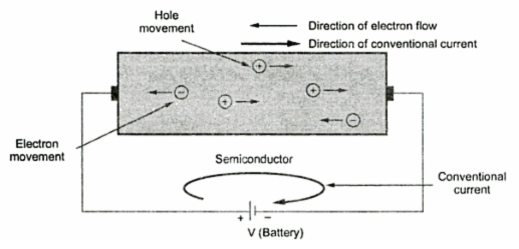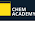### Intrinsic Semiconductors

A sample of semiconductor in its purest from is called an intrinsic semiconductor. The impurity content in intrinsic semiconductor is very very small, of the order of one part in 100 million parts of semiconductor.
1.1 Crystal Structure of Intrinsic Semiconductor
Consider an atomic structure of an intrinsic semiconductor material like silicon. An outermost shell of an atom is capable of holding eight electrons. It is said to be completely filled and stable, if it contains eight electrons. Each of these four electrons from a bond with another valence electron of the neighbouring atoms. This is nothing but sharing of electrons. Such bonds are called covalent bonds. The atoms align themselves to form a three dimensional uniform pattern called a crystal.
The crystal structure of germanium and silicon materials consists of repetitive occurrence in three dimensions of a unit cell. This unit cell is in the form of a tetrahedron with an atom at each vertex. But such a three dimensional structure is very difficult to represent pictorially. Hence a symbolic two dimensional structure is used to represent a three dimensional crystal form, as shown in the Fig. 1(a).
The Fig. 1(a) shows two dimensional representation of a germanium crystal structure. Germanium has a total of 32 electrons. So its first orbit consists of 2 electrons, second consists of 8, third consists of 18 and the valence shell consists of 4 electrons. As there are 4 valence electrons, it is called tetravalent atom.Fig.1 Two dimensional representation of silicon crystal
The covalent bonds are represented by a pair of dotted lines encircling the two electrons forming the covalent bond. The more clear understanding of the covalent bonds can obtained from the Fig. 1(b) which shows the sharing of valence electrons. Both the electrons are shared by the two atoms. Hence the outermost shell of all the atoms is completely filled, and the valence electrons are tightly bound to the parent atoms. No free electrons are available at absolute zero temperature.

1.2 Electrons and Holes in Intrinsic Semiconductors
Let us see what happens at room temperature. At room temperature, the number of valence electrons absorbs the thermal energy, due to which they break the covalent bond and drift to the conduction band. Such electrons become free to move in the crystal as shown in the Fig. 2(a).Fig. 2
Once the electrons are dislodged from the covalent bonds, then they become free. Such free electrons wander in a random fashion in a crystal. The energy required ti break a covalent bond is 0.72 eV for germanium and 1.1 eV for silicon, at room temperature.
When a valence electron drift from valence to conduction band breaking a covalent bond, a vacancy is created in the broken covalent bond. Such a vacancy is called a hole. Whenever an electron becomes free, the corresponding hole gets generated. So free electrons and holes get generated in pairs. The formation of electron-hole pair is shown in the Fig. 2(b) while the corresponding energy band diagram is shown in the Fig. 2(c). Such a generation of electron hole pairs due to thermal energy is called thermal generation.
The concentration of free electrons and holes is always equal in an intrinsic semiconductor. The hole also serves as a carrier of electricity similar to that of free electron.
Key Point : Thus in an intrinsic semiconductors both holes as well as free electrons are the charge carriers.
1.3 Conduction by Electronic and Holes
When battery is applied to an intrinsic material, free electrons move towards positive of battery and responsible for the flow of current. This current is called an electron current. The free election move in conduction band.
Now consider three atoms of Ge in which one of the atom has a hole. Now hole is positively charged. Then valence electron of atom 2 tries to occupy hole of atom 3. When it does so, the hole now gets shifted to atom 2.
Again valence electron of atom 1 fills the hole of atom 2 and hole further moves to atom 1.Fig. 3  Movement of hole
Thus valence electrons move to fill the holes and holes move in opposite direction to valence electrons. The holes keep on moving towards negative of battery. No free electrons are involved in this action.
As holes are positively charged, the movement of holes cause the current called hole current. The hole current is in opposite direction to that of an electron current.

1.4 Conventional Current
The current which flows from positive of battery to the negative of battery, external to the battery is called a conventional current.
It is always the combined effect of electron and hole movement in the semiconductors.
The conventional current direction is opposite to the direction of movement of free electrons while it is same as the direction of movement of holes, in the semiconductors.
Most of the electrons appliances, devices and circuits are analysed using the flow of conventional current.Fig. 4  Conventional current direction opposite to direction of an electron flow
Why intrinsic material are not used ?
The conductivity of an intrinsic semiconductor at room temperature is very small. Very few electron-hole pairs get generated at room temperature. Similarly conductivity due to application of voltage also has very little practical significance. Hence the intrinsic semiconductors in the purest from is not used in practice for manufacturing of electronic devices.
1.5 Conductivity of Intrinsic Semiconductor
The property called conductivity indicates the case with which a material can carry the current. Thus more conductivity means that material can carry high current, very easily. The conductivity of a good conductor is high while that of an insulator is low.
In intrinsic semiconductor, very few electron-hole pairs get generated at room temperature. Hence very small current can be constituted, due to the application of voltage to an intrinsic semiconductor. Thus the conductivity of an intrinsic semiconductor at room temperature is very low/ Such a low conductivity has very little practical significance.
Key Point : Due to low conductivity, the intrinsic semiconductors are not used in practice for manufacturing of electronic devices.
1.6 Recombination of Electronic and Holes
The movement of holes in the valence band is always random and similarly the movement of free electrons in the conduction band is also random. Thermal agitation continues to produce new hole-electron pairs. Occasionally, a free electron approaches a hole and fall into it. This merging of a free electron and a hole is called recombination. After the recombination, an electronic-hole pair gets disappeared. Due to recombination the number of charge carrier decreases. The amount of time between the creation and disappearance of a free electron or hole is called the man life time of the charge carrier.
At any temperature, at any instant, the free electrons and holes, the two types of charge carriers are present in equal numbers. This concentration is called intrinsic concentration. Mathematically this is indicated as,

where       n = Number of free electrons per unit volume
P = Number of holes per unit volume
and           ni = Intrinsic concentration.
The concentration is measured in the units number per m3 or per cm3.

1.